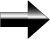Intervals

An interval is a way to specify a part of a number line.  There are several ways of writing intervals.  The easiest is using interval notation.  With interval notation, you simply specify the beginning point and end point and then use the appropriate "bracket" to denote if the end points are included in the interval. A square bracket, "[ ", means the point is included in the interval, and a circle bracket, "(", means the end point is not included in the interval.

An Example:  We want to say that x can be greater than or equal to -5 and less than 10.

Using interval notation, we can write our interval as:   [-5,10)

If we use inequalities, we can write it as: -5 ≤ x < 10

If we use set-builder notation, we write it as {x|-5 ≤ x < 10}, which literally means: “For all x, x is greater than or equal to -5 and less than 10.”

Interval notation is shortest way to write an interval, and it still includes all the important information.

Main Algebra Page